MATLAB Function Referencebesseli

Modified Bessel function of the first kind

Syntax

• ```I = besseli(nu,Z)
I = besseli(nu,Z,1)
[I,ierr] = besseli(...)
```

Definitions

The differential equation

•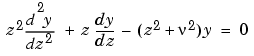where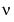is a real constant, is called the modified Bessel's equation, and its solutions are known as modified Bessel functions.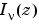and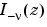form a fundamental set of solutions of the modified Bessel's equation for noninteger.is defined by

•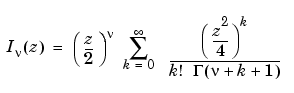where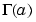is the gamma function.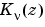is a second solution, independent of. It can be computed using `besselk`.

Description

```I = besseli(nu,Z) ``` computes the modified Bessel function of the first kind,, for each element of the array `Z`. The order `nu` need not be an integer, but must be real. The argument `Z` can be complex. The result is real where `Z` is positive.

If `nu` and `Z` are arrays of the same size, the result is also that size. If either input is a scalar, it is expanded to the other input's size. If one input is a row vector and the other is a column vector, the result is a two-dimensional table of function values.

```I = besseli(nu,Z,1) ``` computes `besseli(nu,Z).*exp(-abs(real(Z)))`.

```[I,ierr] = besseli(...) ``` also returns completion flags in an array the same size as `I`.

 ierr Description `0` `besseli` successfully computed the modified Bessel function for this element. `1` Illegal arguments. `2` Overflow. Returns `Inf`. `3` Some loss of accuracy in argument reduction. `4` Unacceptable loss of accuracy, `Z` or `nu` too large. `5` No convergence. Returns `NaN`.

Examples

Example 1.

• ```format long
z = (0:0.2:1)';

besseli(1,z)

ans =
0
0.10050083402813
0.20402675573357
0.31370402560492
0.43286480262064
0.56515910399249
```

Example 2. `besseli(3:9,(0:.2,10)',1)` generates the entire table on page 423 of  Abramowitz and Stegun, Handbook of Mathematical Functions.

Algorithm

The `besseli` functions uses a Fortran MEX-file to call a library developed by D. E. Amos  .

See Also

`airy`, `besselh`, `besselj`, `besselk`, `bessely`

References

  Abramowitz, M. and I.A. Stegun, Handbook of Mathematical Functions, National Bureau of Standards, Applied Math. Series #55, Dover Publications, 1965, sections 9.1.1, 9.1.89 and 9.12, formulas 9.1.10 and 9.2.5.

  Carrier, Krook, and Pearson, Functions of a Complex Variable: Theory and Technique, Hod Books, 1983, section 5.5.

  Amos, D. E., "A Subroutine Package for Bessel Functions of a Complex Argument and Nonnegative Order," Sandia National Laboratory Report, SAND85-1018, May, 1985.

  Amos, D. E., "A Portable Package for Bessel Functions of a Complex Argument and Nonnegative Order," Trans. Math. Software, 1986.

© 1994-2005 The MathWorks, Inc.